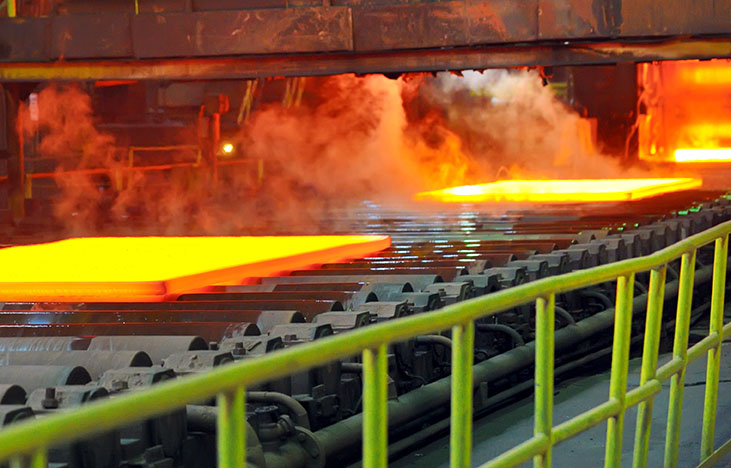# A pinch of Physics is required!You have a piece of aluminium whose mass is $800\text{ g}$, and suppose it is heated up to $1000^\circ\text{C}.$

Now, given that you are cooling it down to $150^\circ\text{C}$, calculate the amount of heat (in Joules) given out during the process of cooling.

Details and assumptions:

• The specific heat capacity of aluminium is $\text{C}_\text{Aluminum}=900 \text{ J}/\text{kg K}.$
• Ignore the heat energy lost to the atmosphere.

×